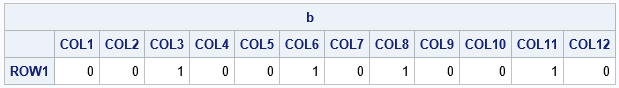In a matrix-vector language such as SAS/IML, it is useful to always remember that the fundamental objects are matrices and that all operations are designed to work on matrices. (And vectors, which are matrices that have only one row or one column.) By using matrix operations, you can often eliminate unnecessary looping over elements of a vector.

Recently, a SAS/IML programmer posted a program that involved a binary (0/1) vector. The program flipped the parity of several elements of the vector. The programmer wrote a loop that iterates over all elements of the vector and flipped the parity of an element if it is in a set of selected indices, as follows:

```proc iml; /* first attempt */ /* 1 2 3 4 5 6 7 8 9 10 11 12 */ b = {0 0 0 1 1 1 0 0 0 1 1 0}; /* a 0/1 binary vector */ FlipIdx = {3 4 5 8 10}; /* flip the parity of b, b, ..., b */ do i = 1 to ncol(b); /* for each element of b */ if element(i, FlipIdx) then do; /* is b[i] in the index? */ if b[i] = 0 then /* if so, flip the parity */ b[i] = 1; else if b[i] = 1 then b[i] = 0; end; end; print b;```In case you are not familiar with the ELEMENT function in SAS/IML, it is similar to the IN operator in the SAS DATA step. It returns a 1 if i is an element of the set FlipIdx and 0 otherwise. Unlike the IN operator, the first argument to the ELEMENT function can be a vector.

The ELEMENT function is very useful, but it is not needed here. Instead of looping over all elements of b, you can simply iterate over the indices in FlipIdx. You can also use the logical negation operator (^) to flip the parity of the elements, as follows:

```/* second attempt */ /* 1 2 3 4 5 6 7 8 9 10 11 12 */ b = {0 0 0 1 1 1 0 0 0 1 1 0}; FlipIdx = {3 4 5 8 10}; do i = 1 to ncol(FlipIdx); /* for each element in the index */ b[FlipIdx[i]] = ^b[FlipIdx[i]]; /* flip the parity */ end; print b;```As indicated earlier, operators in SAS/IML work on matrices and vectors. This includes the negation operator, which operates elementwise on each element of a vector. Specifically, the vector ^v is the negation of the vector v. Consequently, you can eliminate the loop altogether. The program reduces to three lines:

```/* final attempt */ /* 1 2 3 4 5 6 7 8 9 10 11 12 */ b = {0 0 0 1 1 1 0 0 0 1 1 0}; FlipIdx = {3 4 5 8 10}; b[FlipIdx] = ^b[FlipIdx]; /* flip the parity of elements in the set */ print b;```The process of getting rid of DO loops is called vectorization. Experienced SAS/IML programmers are always looking for ways to eliminate loops over rows or columns and replace the loops with vector operations. See the "vectorization" tag of this blog for other vectorization tips.

Share